March 18, 2020

# Deep Tree Network and How to implement a model from a paper

In this post, we are going to implement the neural network from the paper called Deep Tree Learning for Zero-shot Face Anti-Spoofing. The authors of this paper created an architecture based on a decision tree to recognize fake faces and live faces (Face anti-spoofing system).

I suggest you read the paper while reading the post, you can find it here. This post is a little bit long, you can read the first sections related to the math concepts, or skip these sections if you already know these concepts, and then read the code parts.

## Before Starting

This is my first post about implementing neural networks from papers, I will start writing about this to improve my logic when reading papers.

I chose this paper since it contains some math and terms related to linear algebra like the dot product. Even though these concepts are not hard to follow, if you don't know them you won't be able to understand some parts of this paper. Therefore, I will explain these concepts throughout the post. Sometimes reading a paper is hard due to the math it presents, once you know the math, the paper is easier to understand.

This time, the code of the paper is available on GitHub, often this is not the case and the implementation is harder since we don't have a guide. However, the code is quite different from the explanation of the paper so I will implement the network according to the paper and explain the differences with the code. You can find the code on Github here.

## Deep Tree Network (DTN)

The idea behind this network is to have an anti-spoofing system that is able to recognize unknown spoof attacks even if the network wasn't trained on this type of spoof data. In order to achieve this, each node of the tree network learns a different distribution of the spoof data, when known or unknown data arrives, a node called TRU routes this data to the most similar spoof node to make a binary decision (spoof or live face). The training data contains a set of live faces and a set of spoof faces.

This network has three different nodes, a TRU node, a CRU node and a SFL node: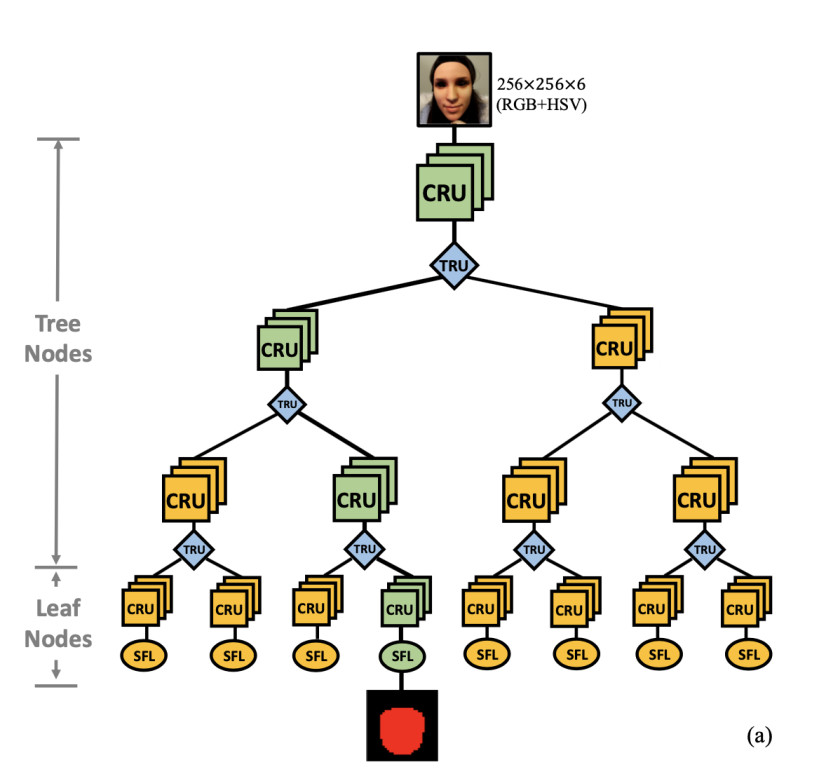Part of Figure 2 from the paper.

In section 3.1.1 of the paper, the authors first talk about a routing function (1) to split the data and a loss function (2) to optimize the parameters of this function, they also mention that this loss function has some problems and may not lead to a good solution.

• The loss can be minimized by increasing the norm of v or x (The norm of a vector is its size or magnitude, we can compute the norm using a distance function like the euclidean distance L2)

Due to the poor routing (1) and loss functions (2) the authors proposed a new routing function (3) where they get rid of the bias term. This routing function computes the dot product between X and v that can be seen as projecting X to the direction of v.

### Dot product

The formula of the dot product is:

``````
A.B

``````

or

``````
|A| x |B| x cos(theta)

``````

Both formulas return the same result, a scalar that represents the position of the vector A projected on the vector B multiplied by the length of B. In the first formula we are multiplying each element of A by each element of B and summing the results: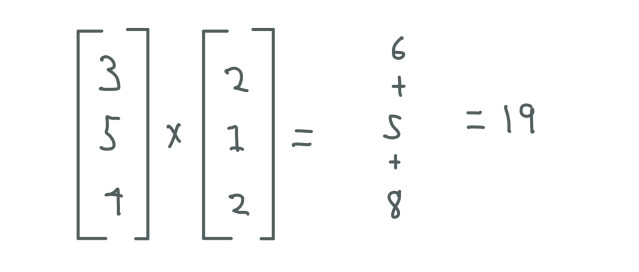Both vectors are represented as column vectors which shape is nx1 where n represents the number of elements. They are called column vectors since we only have one column containing all the elements.

We can see this as first point both vectors in the same direction and then multiply them.

In the second formula, which returns the same result, we multiply the norm (magnitude, size, length) of A, the norm of B and the angle between the two vectors ? (theta).

Certainly, using the first formula is a lot easier since we don't have to compute the norm of each vector or the angle between them. However, the second formula gives us important information about the dot product.

We know that the cosine of some angle returns the position of x in a unit circle: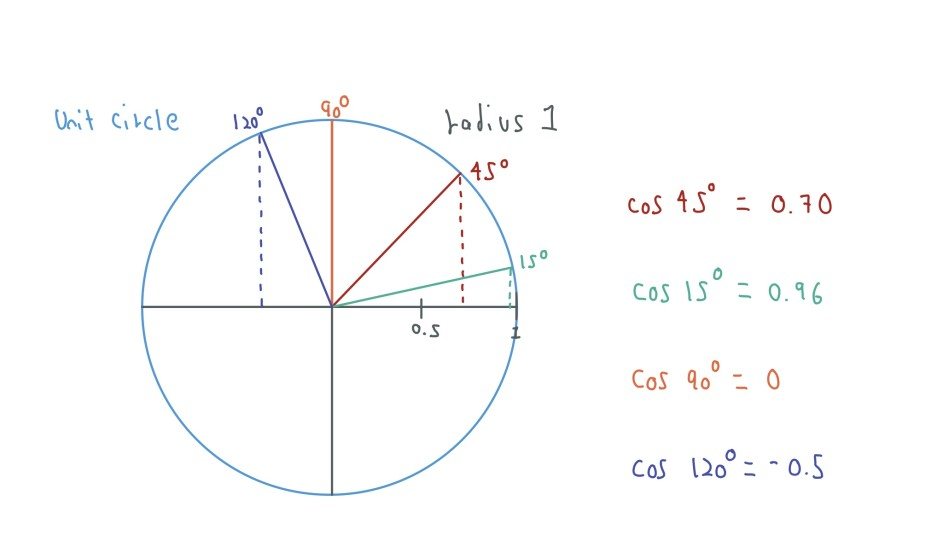In the example above, the cosine of an angle between 0 and 89 returns a positive value, the cosine of 90 returns 0 and the cosine of an angle between 91 and 190 returns a negative value, we can see this as the distance and position of the vector B with respect to the vector A:

• If the value that returns the cosine function is positive and close to 1, both vectors are pointing in the same direction and their distance is small (both vectors are similar).

• If the value that returns the cosine function is positive but close to 0, both vectors are pointing in the same direction and their distance is large (vectors are not similar).

• If the value that returns the cosine function is negative and close to 1 or 0, both vectors are pointing in opposite directions and their distance is large (vectors are completely different).

Now if the value that returns the cosine function is 0 then both vectors are perpendicular or, in vector words, orthogonal (the orange vector and the gray vector). If we return to the second formula we can notice that when we have two orthogonal vectors, the dot product returns 0 since we are multiplying the norm of two vectors and the cosine of the angle between them which is 0. We can see this as projecting the vector A on vector B since both are orthogonal the position of the vector A projected on the vector B is 0.

Therefore, we can also see the dot product as the similarity between two vectors (In fact there is a distance function called cosine similarity that works in the same way computing the dot product).

Now that we know what the dot product is and what represents, we can continue reading the paper. Since we want to project the data X on the vector v, the vector v is designed to obtain the largest variation of data X after projection. Thus, we have a new routing function (3) where now we normalize X and constrain the vector v to have a norm of size 1, we constrain the vector v to avoid the loss function to only increase its size to solve the problem and to force the loss function to find a direction for v that increases the variance (we will see more about this in a moment).

To find the best vector v we can find the largest eigenvector of the covariance matrix of X.

### Eigenvectors

As we have seen, the dot product is the projection of one vector on another vector where we are moving or transforming a vector, matrix multiplication is just the dot product between each row of the first matrix A and each column of the second matrix B. Therefore, matrix multiplication is a transformation of a series of vectors B by a series of transformations A: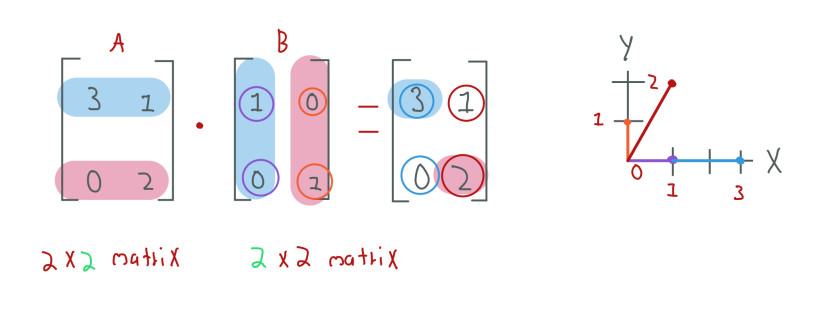In the example above, we have two matrices, A and B, both of shape 2x2 (As we know, the number of columns of the first matrix must equal the number of rows of the second matrix), we can see the elements of the matrix B as two vectors [1, 0] (purple in the graph) and [0, 1] (orange in the graph), after the matrix multiplication we have transformed the vectors of B in [3, 0] (blue in the graph) and [1, 2] (red in the graph). This is the proof that matrix multiplications act as transformations.

From this example we can also notice one more thing, the second vector of B changed its size and position from [0, 1] to [1, 2], however, the first vector, only changed its size, not its position from [1, 0] to [3, 0], this first vector is what we call an eigenvector of matrix A. An eigenvector is a vector that only changes its size but no its position when a transformation is applied. Each eigenvector has an eigenvalue that is the factor by which the eigenvector is scaled, in this case is 3. In this example, the eigenvector increased its size but and eigenvector can also decrease its size.

The paper tells us that finding v is identical to finding the largest eigenvector of the covariance matrix of the data X.

### Covariance Matrix

We can see the variance as the relation between a set of points of the same feature (the height of some population). The covariance is the relation between a set of points of two different features, such as the height and age of some population, while the age increases the height also increases. The covariance matrix gives us information about the covariances between all the features of our data. If our data has two dimensions or two features (height, age) its covariance matrix has a shape of 2x2. We can build a covariance matrix from the matrix data X using a matrix multiplication:

``````
covariance_matrix = np.dot(X.T, X)

``````

Where X.T is our data matrix transposed, if our data has 4 elements and 2 features (4x2) our transposed matrix X.T has a shape of (2x4). When we multiply 2x4 and 4x2 the result matrix, which is the covariance matrix, has a shape of 2x2, we are multiplying each row of the transposed matrix, which now contains all the 4 elements, with each column of our normal matrix X that also contains all the 4 elements.

Basically, we are multiplying all the values of one feature, the height [1.67, 1.75, 1.89, 1.69] by itself and by the other feature, the age [23, 78, 12, 45].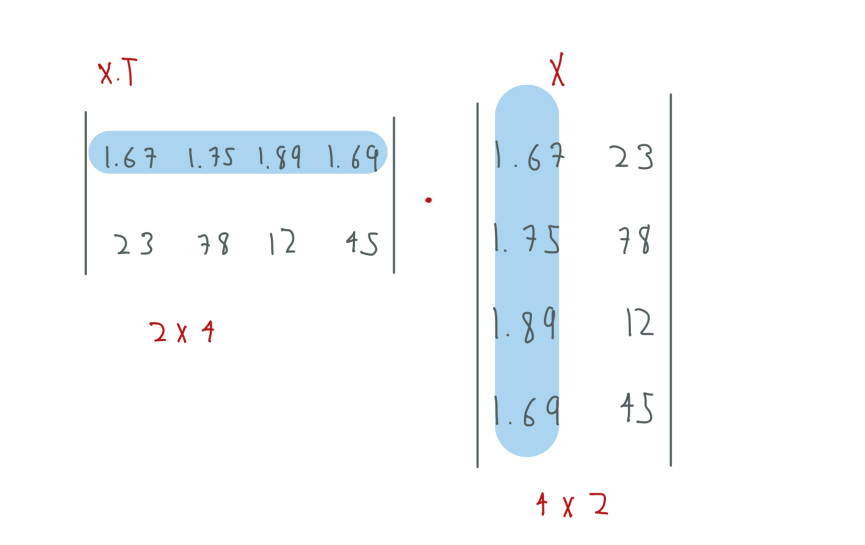The result matrix is: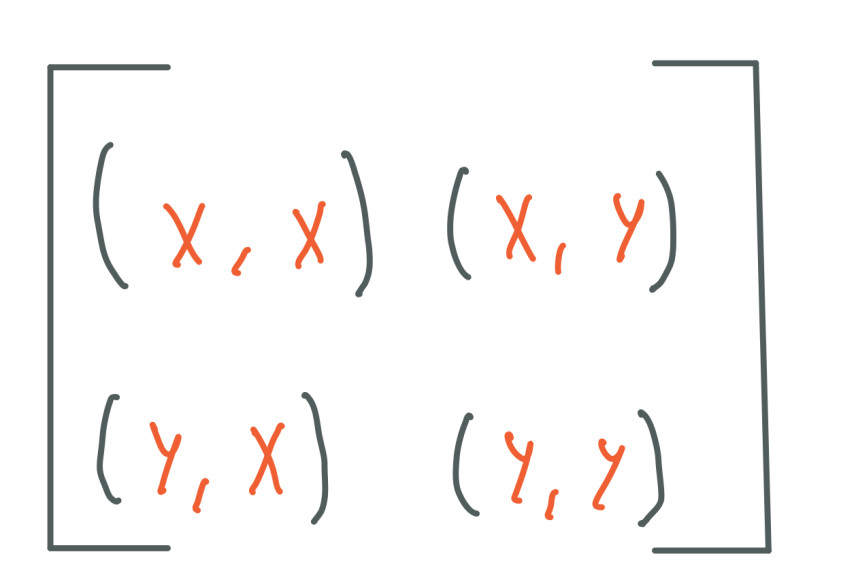Where the diagonal elements are the variances of each feature and the off-diagonal elements are the covariances between features where the covariance of (height = x, age = y) is the same than the covariance of (age = y, height = x).

One step we must perform before the matrix multiplication is normalizing X by subtracting its mean.

To fully understand what the covariance matrix represents, let's examine uncorrelated data of two dimensions where each feature has a variance of 1 and the covariance between the features is 0 (this data is called white data) like the following one: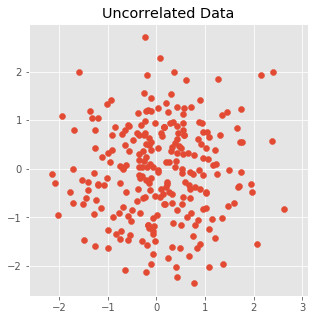The covariance matrix of this data is:

``````
uncorrelated_covariance_matrix = [[1, 0]

[0, 1]]

``````

Now let's see correlated data like the following one: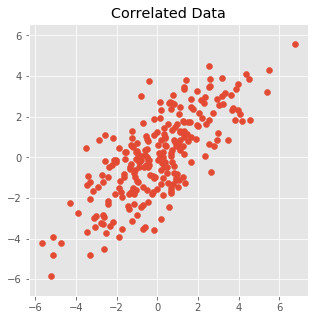The covariance matrix of the correlated data is:

``````
correlated_covariance_matrix = [[[4.25, 3.10]

[3.10, 4.25]]

``````

Where the covariance is 3.10 and both features have the same variance of 4.25.

As we know a matrix multiplication can be seen as a transformation. Thus, we can use the covariance matrix of the correlated data to give form to the uncorrelated data:

``````
transformed_data = X . correlated_covariance_matrix

``````

And the result is: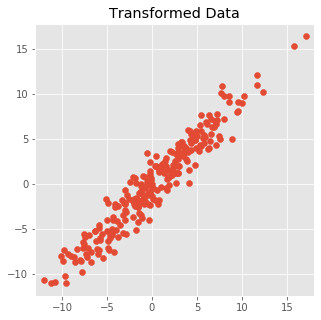Our uncorrelated data now has the same shape and a similar variance and covariance than our correlated data.

Since we can see the covariance matrix as a transformation that provides our data of variance and covariance, then, the covariance matrix also has eigenvectors and eigenvalues. In this case, the biggest eigenvalue and its eigenvector tell us the direction of the largest variance of our data, we want this eigenvector to project our data X on it, this follows a similar workflow than PCA (Here I have a post where I talk about it). So, we could use any vector to project X on it, however, if we use the largest eigenvector then we keep the largest variance between our data.

One more thing I want to talk about related to the covariance matrix is the order in the matrix multiplication, matrix multiplication is not commutative is not the same:

``````
A.B

``````

than

``````
B.A

``````

Usually if we talk about a matrix transforming another matrix we put first the transformation matrix, for example, if we want our covariance matrix to transform a matrix X we would write:

``````
covariance_matrix . X

``````

However, we did this transformation putting first the matrix X:

``````
transformed_data = X . correlated_covariance_matrix

``````

In matrix multiplication the number of columns of the first matrix must match the number of rows of the second matrix, in our case:

``````
X has shape 4x2

correlated_covariance_matrix has shape 2x2

``````

If we want to put first the covariance matrix in the multiplication we have to transpose the data matrix X to match the shapes:

``````
transformed_data = (correlated_covariance_matrix, X.T).T

``````

This gives us the same result since the covariance matrix is symmetric, this means that the off-diagonal elements have the same values, in this case (y,x) and (x, y) are the same.

The covariance matrix has interesting properties that make calculations simpler, for example, the covariance matrix is a square matrix, has the same number of columns and rows, its elements are linearly independent (this means that none of the vectors of a matrix are a linear combination of the rest). Thanks to these properties, we can apply the eigendecomposition to this matrix as we will see in a moment.

Sometimes the order of the matrix multiplication changes in math books and in machine learning books, for instance, is common to see X.T . W in neural networks where we are multiplying our data X by some weights W, this multiplication can also be written as W . X, all depends on the shape of both matrices and how we want to represent our data, this is also applied to the dot product where we can have v . X or x . v.T, this both multiplications mean the same, we are projecting X on v.

### Back to the paper

After the explanation of the covariance matrix we can go back to the paper reading, now we want to optimize the formula (4):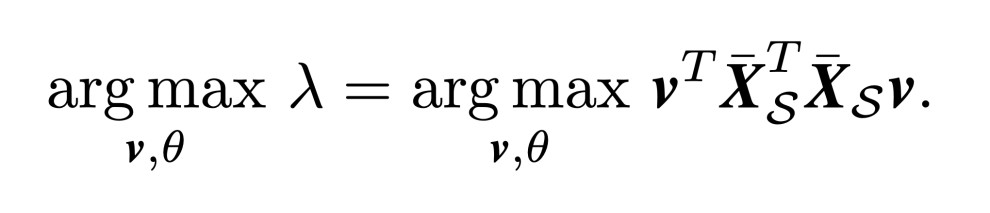In this formula we want to maximize the eigenvalue lambda which is the same than maximize:

``````
v.T(covariance_matrix)v

covariance_matrix = Xs.T . Xs

Xs = X - X.mean()

``````

Here we are using eigendecomposition to re-order the elements and solve for lambda that is the eigenvalue. As we can remember, the eigenvector of a matrix A only changes its size not its position, thus, if we only care about an eigenvector, we can multiply a matrix B by the eigenvalue value and it would be the same than multiply the matrix B by the matrix A since the eigenvector only will change its size and the position does not matter, that's why we have:

``````
(covariance_matrix).v = lambda.v

``````

The eigenvector is affected in the same way if we multiply by the whole covariance matrix or if only multiply by the eigenvalue lambda.

To have the final term we move v that was besides lambda to the other side of the term.

To leave lambda alone we have to move v beside the term:

``````
(covariance_matrix)v

``````

To move v and any other matrix to the other side of one equation we have to compute its inverse, which is written as:

``````
v ^ -1

``````

The inverse of a matrix cancels the effects of the original matrix and the result is the identity matrix which is a matrix full of zeros in the off-diagonal and one's in the main diagonal:

``````
v ^ -1 . v = identity matrix

``````

Compute the inverse matrix is sometimes hard, however, thanks to the properties of the covariance matrix, the transpose of the covariance matrix equals to its inverse:

``````
v.T == v ^ -1

``````

This is the reason why we use the transpose matrix of v at the start of our formula:

``````
v.T(covariance_matrix)v

``````

Finally we have our first loss function (5):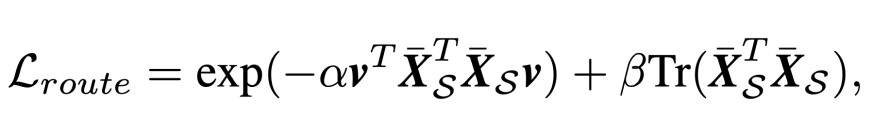This function has two terms, in the first term we are maximizing the eigenvalue. Since we are maximizing this term and we want the loss function to be small, the term should return a value close to 0 if our input is positive and large. We use the exponential function to achieve this, the exponential function returns a small value close to zero if the input is large and negative and returns a large number if the input is positive and large. Due to this, we make the output of the multiplication of the covariance matrix and the eigenvector v negative, then if the eigenvalue is large and positive, the exponential function takes it as negative and returns a small value for the loss function.

The exponential function also limits the optimization. At some input value, the exponential function returns almost the same small number even if we increase the input value by a big factor. Therefore, the loss function will not optimize further the values of v to get a large eigenvalue since it is not necessary.

The second term of this loss function is a regularizer to prevent trivial solutions, this term computes the trace of the covariance matrix, the trace of a matrix returns the sum of its main diagonal elements, in the case of the covariance matrix, its main diagonal elements contain the variances of each feature, computing the trace of the covariance matrix avoid the network to minimize the loss function only increasing the variances of the features of the image.

One trick that we can apply when we don't understand the elements that make up a loss function is to think that the loss function should return a scalar. Thus, we know that the matrix multiplication:

``````
v.T(covariance_matrix)v

``````

and the trace of the covariance matrix:

``````
Tr(covariance_matrix)

``````

Both should return a scalar, as we will see in the code, the shapes of the covariance matrix and the eigenvector v are (1x5120) and (5120x5120) respectively, then when we multiply (1x5120).(5120x5120) the result is (1x5120) that we multiply by the transpose of v (5120x1) which result is (1x1).

With the loss function (5) we will optimize the values of the eigenvector v, v will live inside a custom layer as a trainable variable like weights W and bias b live in normal layers. Therefore, we can see v as a parameter which values will change to optimize the loss function just like weights in any neural network.

As mentioned earlier, to optimize v we have to increase the value of its eigenvalue, this is done by choosing the correct data and by extracting the correct features of this data.

At training time, each TRU node of the network will learn a different distribution, for example, one node will learn the distribution of spoof data where the attack is based on printed pictures, another node could learn the distribution of spoof data where the attack is based on masks that fake users can use to fool the system.

Each TRU node will use a different eigenvector v to learn and keep these distributions. Since these eigenvectors are optimized to point in the direction of maximum variance with respect to its distribution, when new data arrives, this data will be projected to the eigenvector v, if the data is similar to v, the projection will return a value equal or greater than zero due to the fact that both, the eigenvector and the new data are pointing in the same direction. Instead, if the new data is different from the distribution, the projection will return a value less than zero since both, the eigenvector and the new data are pointing in different directions.

We will use the loss function (5) for each TRU node in the network.

In section 3.1.2, the authors mention that to learn correctly the distributions of each node we have to only use the spoof data. Using only the live data (real faces) or a mix of live and spoof data is not enough to learn a good distribution. Thus, we only use the spoof data to build our covariance matrices.

Now, we have our third loss function, the unique loss function (6):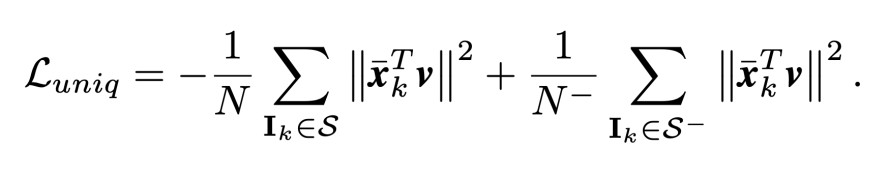Since each node learns a different unique distribution, this loss function suppresses the responses of all the live data along with the spoof data that don't visit this node and is different from the current distribution. This loss function has two terms, in the first term we want to maximize the responses of the spoof data that visit the node (denoted as S), we project each data point of S on the eigenvector v and compute the L2 distance of this projected vector, which we can get squaring the projected vector, this should return large positive numbers denoting that these data points are similar and close to the distribution of that node. In the second term, we have a similar equation, here we compute the same but using the live data and the spoof data that don't visit this node (denoted as S-), in this case, we want small negative responses, denoting that these data points are different from the distribution.

We make the first term negative since we are maximizing its values and the second positive since we are minimizing its values.

We are going to refer to theses two loss functions (5 and 6) as unsupervised loss functions due to the fact we are not using any label. Later we will see two more loss functions which will be known as supervised loss functions.

### Tree Routing Unit (TRU) and Convolutional Residual Unit (CRU)

Now that we know the logic behind the TRU node we will implement it using TensorFlow. First, we need to know the workflow of the Deep Tree Network, each TRU node is connected to a CRU node, the CRU node will always output features of shape (N, H, W, 40) where N is the batch size and H, W are the size of these features.

The input of the neural network is an image of shape (256x256x6) where in the channel axis we have the RGB+HSV color spaces. The first layer of the network is a convolutional layer used to match the channel axis with shape 40:

``````
input_layer = layers.Conv2D(40, (1, 1))(input)

``````

Then the first CRU node takes as input features of shape (N, 256x256x40).

``````
class CRU(tf.keras.Model):

def __init__(self):

super(CRU, self).__init__()

self.conv1 = layers.Conv2D(40, (3, 3), strides=1, padding='SAME', kernel_initializer=initializer, use_bias=False)

self.batch1 = tfa.layers.GroupNormalization(groups=2, axis=3)

self.conv2 = layers.Conv2D(40, (3, 3), strides=1, padding='SAME', kernel_initializer=initializer, use_bias=False)

self.batch2 = tfa.layers.GroupNormalization(groups=2, axis=3)

self.conv3 = layers.Conv2D(40, (3, 3), strides=1, padding='SAME', kernel_initializer=initializer, use_bias=False)

self.batch3 = tfa.layers.GroupNormalization(groups=2, axis=3)

def call(self, x, pooling=True):

model_1 = self.conv1(x)

model_1 = self.batch1(model_1)

model_1 = layers.ReLU()(model_1)

model_1 = x + model_1

model_2 = self.conv2(model_1)

model_2 = self.batch2(model_2)

model_2 = layers.ReLU()(model_2)

model_2 = model_1 + model_2

model_3 = self.conv3(model_1)

model_3 = self.batch3(model_2)

model_3 = layers.ReLU()(model_2)

model = model_3 + model_2

if pooling:

model = layers.MaxPool2D()(model)

return model

``````

CRU Node

In the code above we can see the implementation of the CRU node according to the paper, where we have:

• 3 residual blocks which are initialized using a normal distribution of mean 0 and 0.02 standard deviation, in TensorFlow, we can create an initializer using:
``````
initializer = tf.random_normal_initializer(0., 0.02)

``````
• One pooling layer at the end to reduce the feature space at the half each time (128, 64, 32) (we don't use this pooling layer in the last CRU nodes).

• Group normalization layers that we use instead of the batch normalization layers since the input of each node will have a different number of elements N. Group normalization computes the mean and standard deviation values using a group of elements in the channel axis instead of using the batch axis.

This node has some differences in the published code, first, they only use two residual blocks with two convolutional layers each block, they also use batch normalization layers instead of group normalization layers. I guess this was done because, in the final training, all the CRU nodes take as input all the N batch elements. Thus, we can use batch normalization.

The TRU nodes take as input the extracted features of its connected CRU node:

``````
class TRU(tf.keras.Model):

def __init__(self, filters=20, size=16):

super(TRU, self).__init__()

self.conv = layers.Conv2D(filters, (1, 1), strides=1, padding='SAME', kernel_initializer=initializer)

self.resize = layers.Lambda(lambda layer: tf.image.resize(

layer,

(size, size),

method=tf.image.ResizeMethod.BILINEAR

))

self.reshape = layers.Reshape((size * size * filters,))

self.batch = layers.BatchNormalization(scale=False)

def call(self, x):

model = self.conv(x)

model = self.resize(model)

model = self.reshape(model)

model = self.batch(model)

return model

``````

In this implementation the TRU node code only vectorizes and compresses the input data, the root node compresses the data (X) to a shape of (1x10240) the rest of the TRU nodes compress the data to a shape of (1x5120). Due to the fact that we have compressed X, is easier to compute the covariance matrix and perform the dot product with the eigenvector v. As we can remember, we have to normalize X by subtracting its mean, we can do this using a batch normalization layer without scaling.

A batch normalization layer learns two parameters beta and gamma, the beta parameter centers the data to have mean zero, even though, sometimes it can move the data from the center if it is convenient. The gamma parameter is used to scale the data (make the distribution bigger or smaller), since we only want to remove the mean of the data we "turn off" the gamma parameter.

In the code this is also different, they don't use a batch normalization layer to subtract the mean, they learn the mean value and save it as a trainable variable just like v. In this case, we are following what the paper says.

The TRU node also has to reduce the size of its input value, here I used the tf.image.resize function as a layer but we can also use multiple convolutional or pooling layers to achieve the desired size, in the TRU root node this size is 32x32 and in the rest of the TRU nodes the size is 16x16.

``````
class ProjectionLayer(tf.keras.Model):

def __init__(self, layer_index, input_dim=5120):

super(ProjectionLayer, self).__init__()

self.v = tf.Variable(initial_value=initializer(shape=(input_dim, 1), dtype='float32'), trainable=True, name="v_{}".format(layer_index))

def call(self, compressed_x):

unit_v = self.v / (tf.norm(self.v) + 1e-8)

projection_route = tf.matmul(compressed_x, unit_v)

return projection_route, unit_v

``````

The ProjectionLayer is a custom layer that is in charge of projecting our already vectorized data X (By the TRU code) on the eigenvector v, in this layer we define v as a trainable variable of shape (input_dimx1) in the case of the TRU root node this shape is (10240x1) and in the rest of the nodes the shape is (5120x1), in the call function we constrain v to have a norm of 1 (unit_v, a vector with lengt/norm of 1 is called a unit vector).

In the case of the TRU root node:

``````
input = layers.Input((256, 256, 6))

input_layer = layers.Conv2D(40, (1, 1))(input) # output N, 256, 256, 40

cru_root = CRU(input_layer) # output N, 128, 128, 40

tru_root = TRU(cru_root, filters=10, size=32) # output Nx10240

projection_root_layer = ProjectionLayer(layer_index=1, input_dim=10240)

projection_root, root_unit_v = projection_root_layer(tru_root)

``````

In the code above we have the root level of the DTN:

• A CRU node that extracts features from the data.

• A TRU node that compresses and vectorize these features.

• A ProjectionLayer that project the vectorized data X on an eigenvector v**

The ProjectionLayer returns two variables:

• projection_root is an array of shape Nx1 that contains the projection result of each data point, this result is zero or a positive number if the data point is similar to the distribution of the layer or a negative number if the data point is different.

• root_unit_v is the eigenvector v of this layer, we need this vector to compute the loss function.

To end this section we are going to look at the implementation of the route loss function (5) and the unique loss function (6):

``````
def compute_tru_loss(spoof_x, not_x, unit_v, training):

loss = tf.Variable(0.0, dtype=tf.float32)

if training and tf.shape(spoof_x) > 0:

transpose_unit_v = tf.transpose(unit_v, [1, 0])

covariance_matrix = tf.matmul(spoof_x, spoof_x, transpose_a=True)

trace = tf.linalg.trace(covariance_matrix)

eigenvalue = tf.matmul(tf.matmul(transpose_unit_v, covariance_matrix), unit_v)

route_loss = tf.exp(-alpha * eigenvalue) + beta * trace

spoof_x_loss = -tf.reduce_mean(tf.square(tf.matmul(spoof_x, unit_v)))

not_x_loss = tf.reduce_mean(tf.square(tf.matmul(not_x, unit_v)))

unique_loss = spoof_x_loss + not_x_loss

loss = (a3 * route_loss) + (a4 * unique_loss)

return loss

``````

tf.shape is used to obtain the shape of a TensorFlow matrix.

If the TRU node didn't receive data then we can't build the covariance matrix either compute its loss function.

We know that in order to build the covariance matrix, we only have to use the spoof data that visit this node and to compute the unique loss we need the live data and the spoof data that don't visit this node. Thus, the compute_tru_loss function takes as input the split data (spoof_x, not_x) and the eigenvector v of the previous ProjectionLayer, we split the data into spoof_x (data that is similar to the distribution of v) and into not_x (the rest of the data). We split the data using the projection_root, if the data point is similar (a value of 0 or greater) then it goes to the spoof_x array, otherwise (a negative value) the data point goes to the not_x variable.

This function is easy to understand if you look at the written loss functions, the a3 and a4 variables are regularizers which values are:

``````
a3 = 2.0

a4 = 0.001

``````

The most important part of this function is the eigenvalue computation:

``````
eigenvalue = tf.matmul(tf.matmul(unit_v, covariance_matrix), transpose_unit_v)

``````

We want v (unit_v) to have a large corresponding eigenvector.

### Supervised Loss Functions

In section 3.2 of the paper, the authors present two more loss functions that are related to our last node SFL (Supervised Feature Learning). This node is used as a leaf node and connected to the last CRU nodes. SFL has two outputs, the first is a binary classifier (1: spoof face, 0: live face), the second is a binary mask of shape (32x32x1) which pixels values are one's if they are spoofing mediums.

The implementation of this node is quite simple:

``````
class SFL(tf.keras.Model):

def __init__(self):

super(SFL, self).__init__()

self.conv1 = layers.Conv2D(1, (1, 1), activation="sigmoid", kernel_initializer=initializer)

self.conv2 = layers.Conv2D(40, (3,3), kernel_initializer=initializer)

self.conv3 = layers.Conv2D(40, (3,3), kernel_initializer=initializer)

self.flat = layers.Flatten()

self.dense1 = layers.Dense(500, kernel_initializer=initializer)

self.dense2 = layers.Dense(1, activation="sigmoid", kernel_initializer=initializer)

def call(self, x):

classification = self.conv2(x)

classification = self.conv3(classification)

classification = self.flat(classification)

classification = self.dense1(classification)

classification = self.dense2(classification)

``````

The SFL node takes as input the output of the last CRU node, this output has a shape of (N, 32, 32, 40).

The loss functions are also simple, the first one (7, 8) is just the cross-entropy loss (we can use the binary cross-entropy as well):

``````
binary_cross_entropy = tf.keras.losses.BinaryCrossentropy()

``````

The second loss function (9) is just the L1 distance between the true mask and the predicted mask:

``````
L1_loss = tf.keras.losses.MeanAbsoluteError()

``````

The code to compute both loss functions is the following one:

``````

total_loss = tf.Variable(0.0, dtype=tf.float32)

data_in_node = tf.reduce_sum(tf.cast(mask, tf.float32))

if data_in_node > 0:

total_loss += (a1 * classification_loss) + (a2 * mask_loss)

``````

We have to compute the two loss functions for each SFL node, for this reason, we need the for loop. Inside the compute_sfl_loss function we have several variables:

• classes_pred: This is a list with 8 elements (one element by array), each element has a shape of (Nx1) where N is the size of the batch size.

• masks_pred: A list with 8 elements, each element has a shape of (Nx32x32x1).

• labels: These are the labels of the true classes, is an array of shape (Nx1).

• masks_true: These are the true masks, is an array of shape (Nx32x32x1).

Inside the for loop, first, we have to count the number of data points (images) that arrived at the SFL node, we only compute the loss for that node if we have data points, we use the boolean variable masks of shape (Nx1) that contains True if the data point arrived at the node or False otherwise. Since we only want to compute the loss with respect to the elements that arrived at the node, we use the masks variable to mask the other variables (labels, classes_pred, masks_pred), after this masking the variables (masked_labels, masked_classes, masked_mask) only contain the elements of this node. a1 and a2 are regularization coefficients which values are:

``````
a1 = 0.001

a2 = 1.0

``````

One important note, In the code on Github the authors used the L1 loss to compute both loss functions, the classification and mask loss, but also since they used a different masking method, in these loss functions even if the node didn't receive data points the loss function is computed but reduced to a really small number close to zero, thanks to this, the weights of this node have small updates which could help to the training, in our case the weights don't receive updates.

The overall loss (10) contains the 4 loss functions that we have seen so far.

## DTN implementation

Finally, we will see how we can implement this tree network along with all its nodes. Considering the fancy architecture, we could easily get lost, for this reason, we will use the following image to have a visual representation of the network: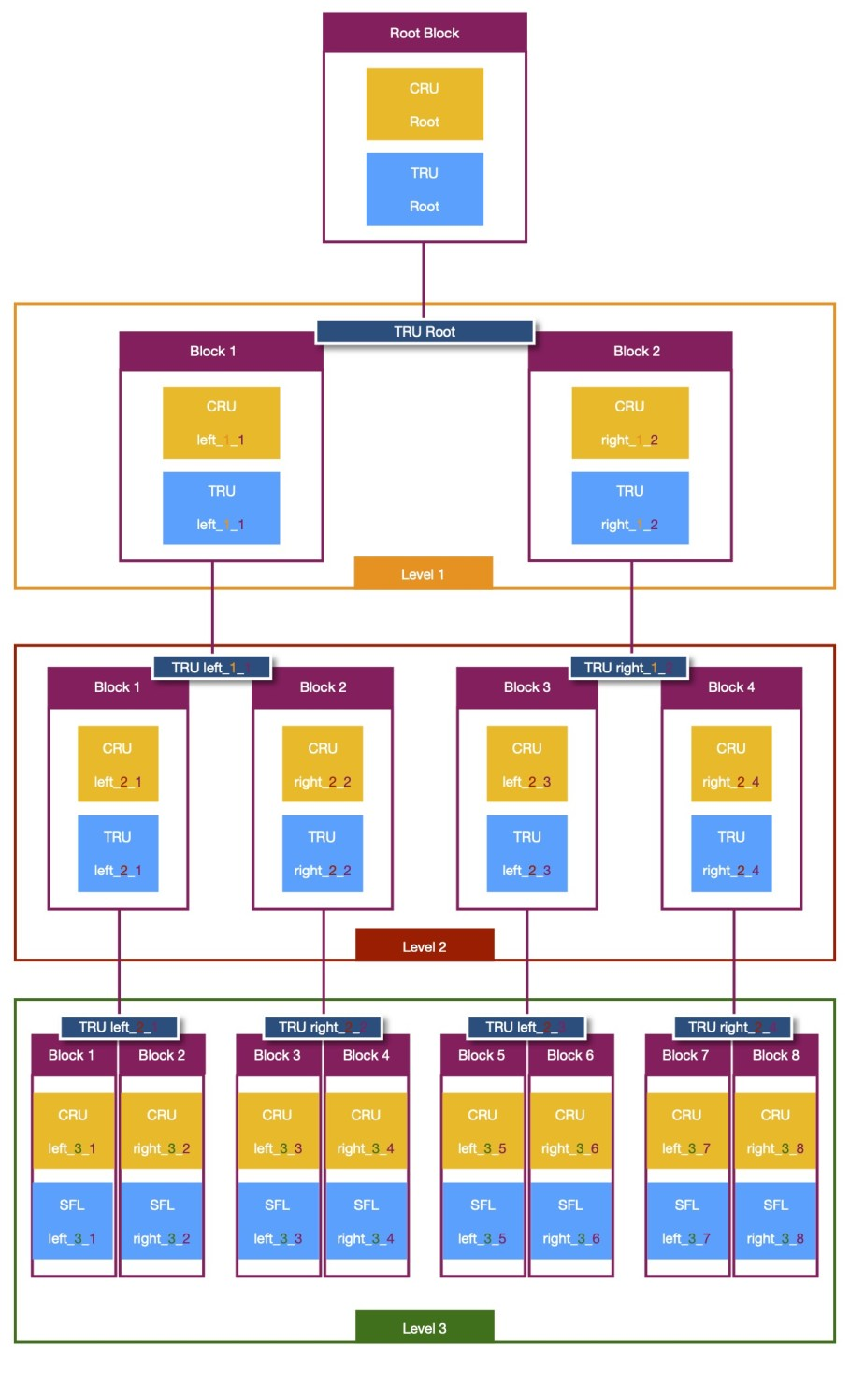Each node, except for the root nodes, has the following nomenclature:

``````
name_position_level_block

``````

We have 3 levels (4 if we count the root as a level), each pair of (CRU, TRU) or (CRU, SFL) nodes count as a block. Since we split by two each level, we have 2, 4, 8 blocks for each level. The dark blue rectangle above each block indicates the TRU node that is used to split the data for the left and right nodes.

Here we can see the complete code to create the network:

``````
@tf.function

def call(self, x, labels, training=True):

input_layer = self.input_layer(x)

# Root Level

cru_root = self.cru_1(input_layer)

tru_root = self.tru_1(cru_root)

projection_root, root_unit_v = self.projection_root_layer(tru_root)

if training:

spoof_mask = tf.where(labels == 1, x=True, y=False)

live_mask = tf.where(labels == 0, x=True, y=False)

else:

right_data = np.array([])

left_data = np.array([])

unsupervised_root_loss = compute_tru_loss(right_data, left_data, root_unit_v, training)

# Level 1 ================================================================================================================================

# Block 1, 2 Create masks

right_mask = tf.where(projection_root >= 0, x=True, y=False)

left_mask = tf.where(projection_root < 0, x=True, y=False)

# Block 1 (left node)

cru_left_1_1 = self.cru_2(cru_root)

tru_left_1_1 = self.tru_2(cru_left_1_1)

projection_left_1_1, left_1_1_unit_v = self.projection_left_1_1_layer(tru_left_1_1)

# Block 1 Masking

unsupervised_left_1_1_loss = compute_tru_loss(left_data, right_data, left_1_1_unit_v, training)

# Block 2 (right node)

cru_right_1_2 = self.cru_3(cru_root)

tru_right_1_2 = self.tru_3(cru_right_1_2)

projection_right_1_2, right_1_2_unit_v = self.projection_right_1_2_layer(tru_right_1_2)

# Block 2 Masking

unsupervised_right_1_2_loss = compute_tru_loss(right_data, left_data, right_1_2_unit_v, training)

# Level 2 ===============================================================================================================================

# Block 1, 2 Create masks

right_mask = tf.where(projection_left_1_1 >= 0, x=True, y=False)

left_mask = tf.where(projection_left_1_1 < 0, x=True, y=False)

# Block 1 (left node)

cru_left_2_1 = self.cru_4(cru_left_1_1)

tru_left_2_1 = self.tru_4(cru_left_2_1)

projection_left_2_1, left_2_1_unit_v = self.projection_left_2_1_layer(tru_left_2_1)

# Block 1 Masking

unsupervised_left_2_1_loss = compute_tru_loss(left_data, right_data, left_2_1_unit_v, training)

# Block 2 (right node)

cru_right_2_2 = self.cru_5(cru_left_1_1)

tru_right_2_2 = self.tru_5(cru_right_2_2)

projection_right_2_2, right_2_2_unit_v = self.projection_right_2_2_layer(tru_right_2_2)

# Block 2 Masking

unsupervised_right_2_2_loss = compute_tru_loss(right_data, left_data, right_2_2_unit_v, training)

# Block 3, 4 Create masks

right_mask = tf.where(projection_right_1_2 >= 0, x=True, y=False)

left_mask = tf.where(projection_right_1_2 < 0, x=True, y=False)

# Block 3 (left node)

cru_left_2_3 = self.cru_6(cru_right_1_2)

tru_left_2_3 = self.tru_6(cru_left_2_3)

projection_left_2_3, left_2_3_unit_v = self.projection_left_2_3_layer(tru_left_2_3)

# Block 3 Masking

unsupervised_left_2_3_loss = compute_tru_loss(left_data, right_data, left_2_3_unit_v, training)

# Block 4 (right node)

cru_right_2_4 = self.cru_7(cru_right_1_2)

tru_right_2_4 = self.tru_7(cru_right_2_4)

projection_right_2_4, right_2_4_unit_v = self.projection_right_2_4_layer(tru_right_2_4)

# Block 4 Masking

unsupervised_right_2_4_loss = compute_tru_loss(right_data, left_data, right_2_4_unit_v, training)

# Level 3 ===============================================================================================================================

# Block 1 (left node)

cru_left_3_1 = self.cru_8(cru_left_2_1, pooling=False)

class_left_3_1, map_left_3_1 = self.sfl_1(cru_left_3_1)

mask_left_3_1 = tf.where(projection_left_2_1 < 0, x=True, y=False)

# Block 2 (right node)

cru_right_3_2 = self.cru_9(cru_left_2_1, pooling=False)

class_right_3_2, map_right_3_2 = self.sfl_2(cru_right_3_2)

mask_right_3_2 = tf.where(projection_left_2_1 >= 0, x=True, y=False)

# Block 3 (left node)

cru_left_3_3 = self.cru_10(cru_right_2_2, pooling=False)

class_left_3_3, map_left_3_3 = self.sfl_3(cru_left_3_3)

mask_left_3_3 = tf.where(projection_right_2_2 < 0, x=True, y=False)

# Block 4 (right node)

cru_right_3_4 = self.cru_11(cru_right_2_2, pooling=False)

class_right_3_4, map_right_3_4 = self.sfl_4(cru_right_3_4)

mask_right_3_4 = tf.where(projection_right_2_2 >= 0, x=True, y=False)

# Block 5 (left node)

cru_left_3_5 = self.cru_12(cru_left_2_3, pooling=False)

class_left_3_5, map_left_3_5 = self.sfl_5(cru_left_3_5)

mask_left_3_5 = tf.where(projection_left_2_3 < 0, x=True, y=False)

# Block 6 (right node)

cru_right_3_6 = self.cru_13(cru_left_2_3, pooling=False)

class_right_3_6, map_right_3_6 = self.sfl_6(cru_right_3_6)

mask_right_3_6 = tf.where(projection_left_2_3 >= 0, x=True, y=False)

# Block 7 (left node)

cru_left_3_7 = self.cru_14(cru_right_2_4, pooling=False)

class_left_3_7, map_left_3_7 = self.sfl_7(cru_left_3_7)

mask_left_3_7 = tf.where(projection_right_2_4 < 0, x=True, y=False)

# Block 8 (right node)

cru_right_3_8 = self.cru_15(cru_right_2_4, pooling=False)

class_right_3_8, map_right_3_8 = self.sfl_8(cru_right_3_8)

mask_right_3_8 = tf.where(projection_right_2_4 >= 0, x=True, y=False)

# Outputs

classes_pred = [class_left_3_1, class_right_3_2, class_left_3_3, class_right_3_4,

class_left_3_5, class_right_3_6, class_left_3_7, class_right_3_8]

maps_pred = [map_left_3_1, map_right_3_2, map_left_3_3, map_right_3_4,

map_left_3_5, map_right_3_6, map_left_3_7, map_right_3_8]

unsupervised_loss = unsupervised_root_loss + unsupervised_left_1_1_loss + unsupervised_right_1_2_loss

unsupervised_loss += unsupervised_left_2_1_loss + unsupervised_right_2_2_loss + unsupervised_left_2_3_loss

unsupervised_loss += unsupervised_right_2_4_loss

if training:

return classes_pred, maps_pred, masks, unsupervised_loss

else:

return classes_pred, maps_pred, masks

``````

From the code above we can notice some things:

• As we have seen, we divide the work of the TRU node into two, TRU compresses the data, ProjectionLayer project this compressed data on v.

• The ProjectionLayer returns the projection results which we use to create the masks and split the data to feed the nodes of the next level.

• Even though all the CRU, TRU and SFL nodes take the complete batch of data N, the loss function (compute_tru_loss) takes into account the split of the data by the previous ProjectionLayer, TRU node. With this, even if the TRU node compressed the complete batch we only use the right data to compute the covariance matrix and as S in the unique loss function.

The name of the split data is right data and left data, the right data are the elements that are similar to the distribution of v (which values are equal or greater than 0), the left data are the elements that are different to the distribution of v (which values are less than zero). This can be a little bit confusing since this doesn't indicate to which node the data should go.

The root TRU node splits the data based on the true labels of spoof data. Thus, the v of the root TRU learns the distribution of the spoof data, then, the right node of the next level (right_1_2) takes as input the right data to build the covariance matrix and used as S and the left data is used as S-, whereas, the left node of the next level (left_1_1) takes as input the left data to build the covariance matrix and used as S and the right data is used as S-.

In other words, the current right and left nodes use the split data of the previous TRU nodes, where the right nodes take as input the right data to learn from it, the left nodes take as input the left data to learn from it.

Since this could be a little bit confusing, the right and left data that is used in the loss function for each node is not the data that is similar to the v of that node, but the data that is similar to the data of the previous v.

In the last part of the code we return as output the classes predicted, the maps predicted (The masks that indicate the areas of the spoof data), the total loss of the TRU nodes and the masks to know what data points arrived at the SFL nodes.

### Training

In order to train this network we use a learning rate of 0.001, a batch size of 32 and train for 15 epochs as section 5.1 (Parameter setting) indicates. At the end of section 3.3 we are also told to train the TRU nodes and CRU/SFL nodes alternately keeping the parameters of the TRU nodes fixed while training the CRU/SFL nodes and vice versa. However, in the code on GitHub the authors first trained the CRU/SFL for 10,000 steps and then trained all the nodes together.

This last training is easier to perform, if we only want to compute the loss of some nodes we compute the gradients with respect to the loss function of only those nodes, if we want to compute the loss of all the nodes we take into account all the nodes' loss functions to compute the gradients. In our DTN this is easy to perform since we have two loss functions, if we want to train only the CRU/SFL nodes we only use the supervised loss to compute the gradients. Let's see how this is quite simple in the training code:

``````
@tf.function

def train_step(images, labels, masks_true, total_steps):

with tf.GradientTape() as tape:

classes_pred, maps_pred, masks, unsupervised_loss = model(images, labels, training=True)

supervised_loss = compute_sfl_loss(labels, masks_true, classes_pred, maps_pred, masks)

if total_steps > 10000:

loss = supervised_loss + unsupervised_loss

else:

loss = supervised_loss

return loss

def train(epochs, total_steps):

for epoch in range(epochs):

batch_time = time.time()

epoch_time = time.time()

step = 0

epoch_count = f"0{epoch + 1}/{epochs}" if epoch < 9 else f"{epoch + 1}/{epochs}"

# Change for a true generator

for images, labels, masks_true in zip(images_batches, labels_batches, masks_batches):

loss = train_step(images, labels, masks_true, total_steps)

loss = float(loss.numpy())

step += 1

print('\r', 'Epoch', epoch_count, '| Step', f"{step}/{train_steps}",

'| loss:', f"{loss:.5f}", "| Step time:", f"{time.time() - batch_time:.2f}", end='')

batch_time = time.time()

total_steps += 1

loss_results.append(loss)

checkpoint.save(file_prefix=checkpoint_prefix)

print('\r', 'Epoch', epoch_count, '| Step', f"{step}/{train_steps}",

'| loss:', "| Epoch time:", f"{time.time() - epoch_time:.2f}")

``````

In the train_step function if we have been training the network for more than 10,000 steps we add the unsupervised loss to the total loss. Thus, we update all the nodes. It's important to make this addition inside the tf.GradientTape() scope to compute the gradients correctly.

If you want to see the complete code, check here. I didn't train the neural network but if you can get the training data and have a good machine you can give it a try, I only used dummy data to confirm that the code works.

### Inference

The paper neither the code is explicit about how to use the network for inference. However, we can assume that if we pass only one image through the network, this image will arrive at all the leaf nodes SFL but we should take the predicted class of only one of those nodes.

This could not perform well since the image has to go through all the nodes. Instead, we could take the trained network and create a new model where the nodes only take the image if needed.

## Final thoughts

This paper was interesting to read. You can realize how relevant math is when reading some papers. Even if the math is not hard to understand, you can have a hard reading when you don't know it.

Most of the time, authors state that we already know the math in the papers and don't explain further. This is comprehensible considering that explaining everything would take a lot of time and space. Thus, we should search for the concepts we don't understand while reading papers.